2019-12-23 19:04:57 weixin_44244154 阅读数 30
• ###### MATLAB图像处理

全面系统的学习MATLAB在图像处理中的应用

19905 人正在学习 去看看 魏伟

# 实验内容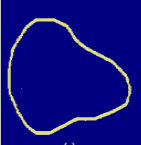# 实验原理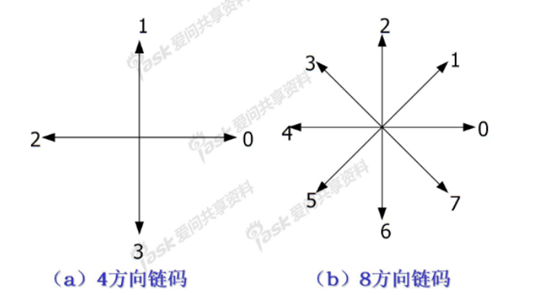可以按照各个方向的数字来确定相应的图像边界的方向，从而构成图像，对于每相邻的两个方向之间的差则构成了一阶差分码，一阶差分码的最小值即形状数。

## 过程分析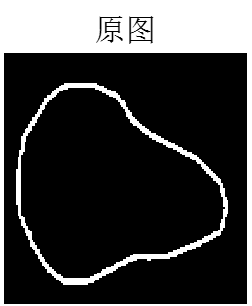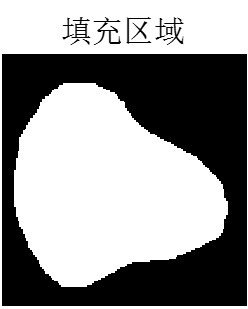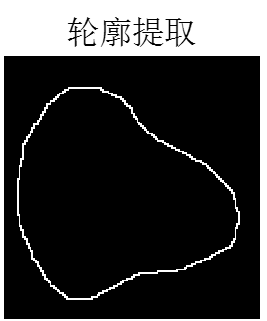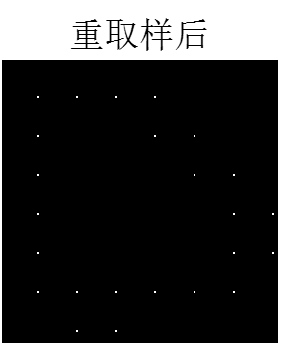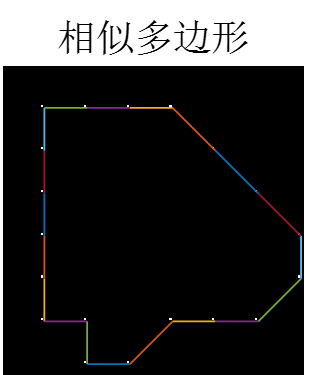# 实验代码如下：

clear all
clc
t1=im2bw(t1);%图像二值化
t=t1;
%图像边缘有白边，将其过滤掉
t(:,1)=0;
t(144:145,:)=0;
figure;
imshow(t),title('原图');
[m,n]=size(t);%获取图像大小
%%
%按每一行来进行扫描，找出图像每一行白色区域的临界端点，并将中间区域填充为白色
for i=1:m
lie=t(i,:);
x1=0;x2=n;
zong=sum(lie);
if zong~=0
for j=1:n
if lie(j)==1
x1=j;
break
end
end
lie=flip(lie);
for j=1:n
if lie(j)==1
x2=n-j+1;
break
end
end

for k=x1+1:x2-1
t(i,k)=1;      %将中间区域填充为白色
end
end
end
figure;
imshow(t),title('填充区域');
t=bwperim(t,8);%对二值图像进行轮廓提取
figure;
imshow(t),title('轮廓提取');
%%
%重采样
t1=t;
t2=zeros(m,n);
for i=1:m
for j=1:n
if t(i,j)==1
if round(j/20)==0
t2(20*round(i/20),20*(round(j/20)+1))=1;
else
t2(20*round(i/20),20*round(j/20))=1;
end
end
end
end
figure,imshow(t2),title('重取样后');
%%
qidian=[0 0];%保存起点
lianma=[];%保存链码
duandian=zeros(20,2);%保存端点
k=0;
t=t2;

%找出一个起点进行链码搜索
for i=1:m
for j=1:n
if t(i,j)==1
qidian=[i j];
qidian
break;
end
end
end

x=qidian(1);
y=qidian(2)+1;

while (x~=qidian(1)||y~=qidian(2)) && k<21
k=k+1;
if k==1
y=y-1;
end

if y+20<=n
if t(x,y+20)==1
lianma(k)=0;
duandian(k,1)=x;
duandian(k,2)=y;
t(x,y)=0;
y=y+20;
continue;
end
end

if x-20>0&&y+20<=n
if t(x-20,y+20)==1
lianma(k)=1;
duandian(k,1)=x;
duandian(k,2)=y;
t(x,y)=0;
x=x-20;
y=y+20;
continue;
end
end

if x-20>0
if t(x-20,y)==1
lianma(k)=2;
duandian(k,1)=x;
duandian(k,2)=y;
t(x,y)=0;
x=x-20;
continue;
end
end

if x-20>0&&y-20>0
if t(x-20,y-20)==1
lianma(k)=3;
duandian(k,1)=x;
duandian(k,2)=y;
t(x,y)=0;
x=x-20;
y=y-20;
continue;
end
end

if y-20>0
if t(x,y-20)==1
lianma(k)=4;
duandian(k,1)=x;
duandian(k,2)=y;
t(x,y)=0;
y=y-20;
continue;
end
end

if y-20>0
if t(x+20,y-20)==1
lianma(k)=5;
duandian(k,1)=x;
duandian(k,2)=y;
t(x,y)=0;
x=x+20;
y=y-20;
continue;
end
end

if t(x+20,y)==1
lianma(k)=6;
duandian(k,1)=x;
duandian(k,2)=y;
t(x,y)=0;
x=x+20;
continue;
end

if y+20<=141
if t(x+20,y+20)==1
lianma(k)=7;
duandian(k,1)=x;
duandian(k,2)=y;
t(x,y)=0;
x=x+20;
y=y+20;
continue;
end
end
end
lianma
xt=t2-t;
figure,imshow(xt),title('相似多边形'),hold on;
for i=1:19
if i==19
plot([duandian(19,2);duandian(1,2)],[duandian(19,1);duandian(1,1)]);
else
plot(duandian(i:i+1,2),duandian(i:i+1,1));
end
end



2019-02-18 21:29:30 dzm123lalala 阅读数 105
• ###### MATLAB图像处理

全面系统的学习MATLAB在图像处理中的应用

19905 人正在学习 去看看 魏伟

# 形态学基本概念

1. 对图像进行观察和处理，从而达到改善图像质量的目的
2. 米哦啊书和定义图像的各种几何参数和特征，如面积，周长，联通度，颗粒度，骨架和方向性等。

## 几个概念

$A^C=\{w|w \notin A\}$

$A-B=\{w|w\in A,w \notin B \}=A \cap B^C$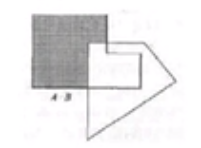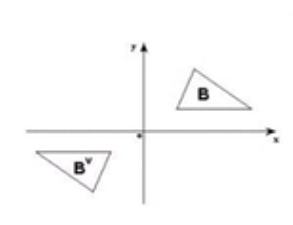$(A)_z=\{c|c=a+z,a \in A\}$

# 腐蚀与膨胀

## 膨胀

$A\oplus B=\{z|(\hat{B})_z \cap A \neq \Phi\}$
B称为结构元素。结构元素先进行反射，之后进行z平移，之后和A进行交运算，若击中，记录下平移量z。

$D_B(A)= A\oplus B=\{a|B_a\uparrow A\}$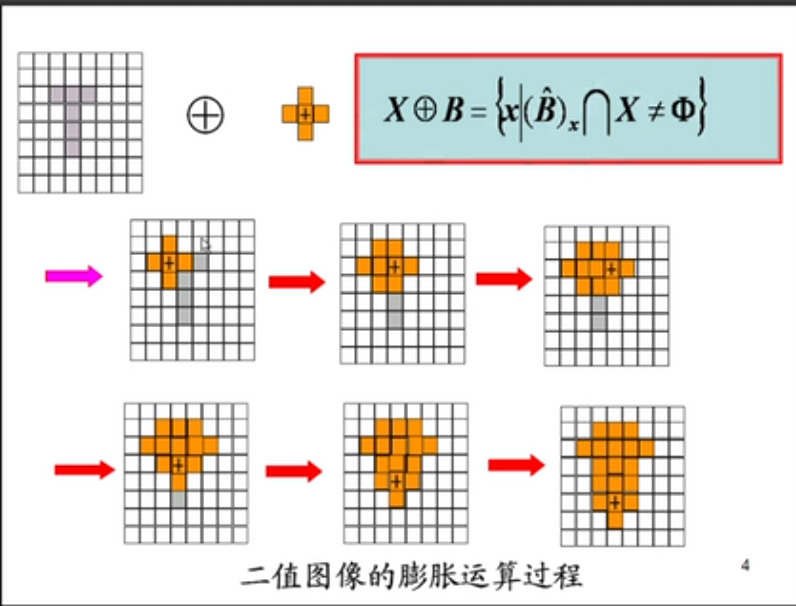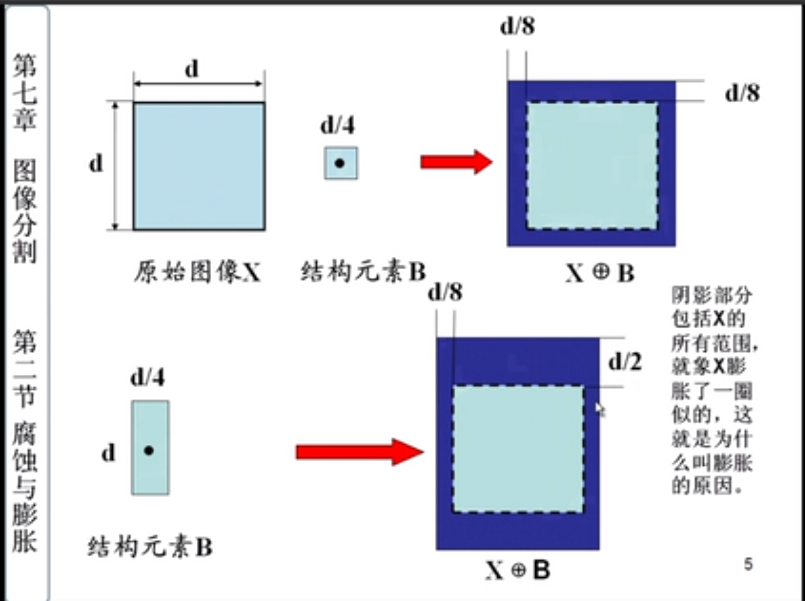## 腐蚀

$A\circleddash B=\{z|(B)_z \subseteq A\}$

$E_B(A)=A\circleddash B=\{a|B_a \subset A\}$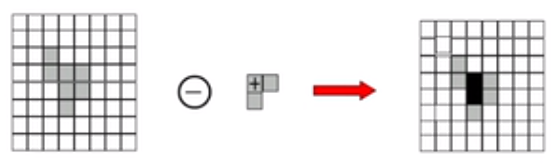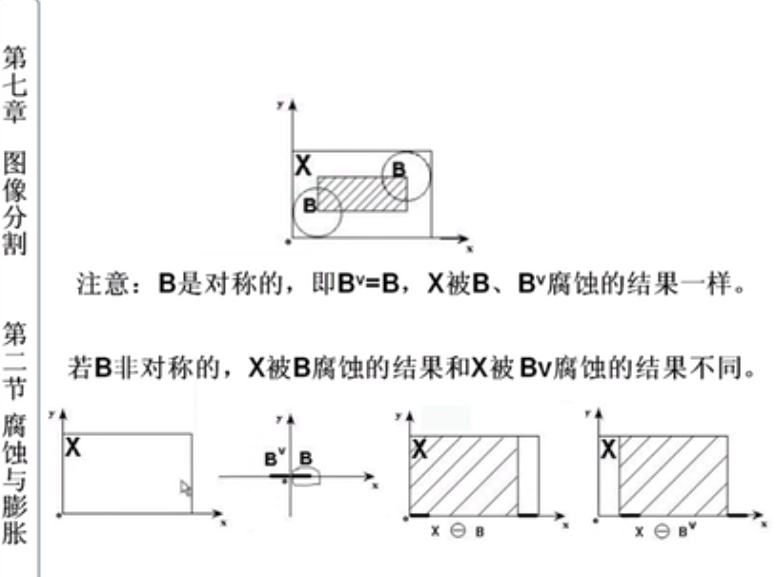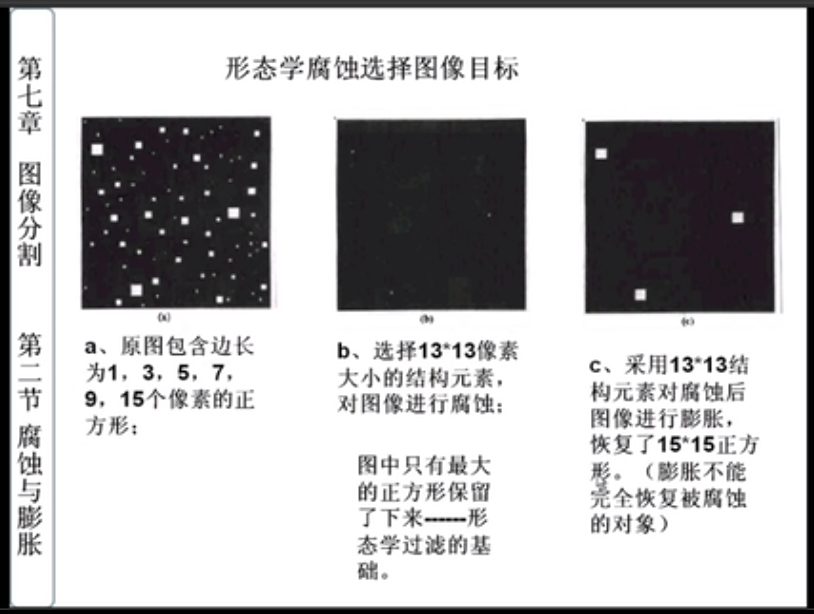## 膨胀和腐蚀的性质

• 膨胀和腐蚀运算的对偶性
$(X\circleddash B)^C=X^C \oplus \hat{B} \quad \quad (X\oplus B)^C=X^C \circleddash \hat{B}$
X对B的腐蚀运算的补集等于X的补集对B的反射的膨胀运算；
X对B的反射运算的补集等于X的补集对B的腐蚀的膨胀运算。
河岸的补集为河面，对河岸的腐蚀等价于对河面进行膨胀。

• 膨胀运算具有互换性，腐蚀运算不具有互换性。
$(X\oplus B)\oplus A=(X\oplus A)\oplus B$
$(X\circleddash B)\circleddash A \neq (X\circleddash A)\circleddash B$

• 腐蚀和膨胀具有组合性。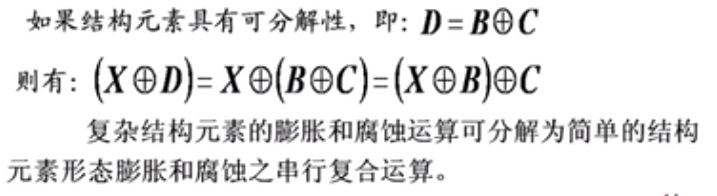# 开运算与闭运算

## 开运算

$A \circ B=(A\circleddash B)\oplus B$

• 消除细小对象
• 在细小粘连处分离对象
• 在不改变形状的前提下，平滑对象的边缘。

$3\times 3$$5\times 5$的矩形结构元素分别进行开运算：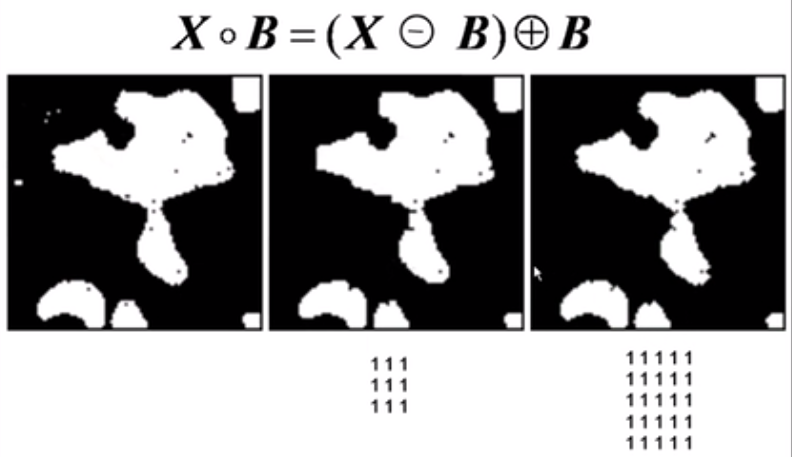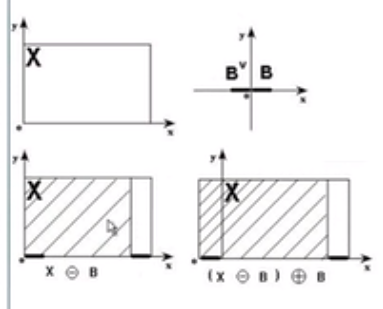## 闭运算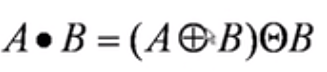• 填充对象内细小空洞
• 链接接近的对象
• 再不明显改变面积的前提下，平滑对象的边缘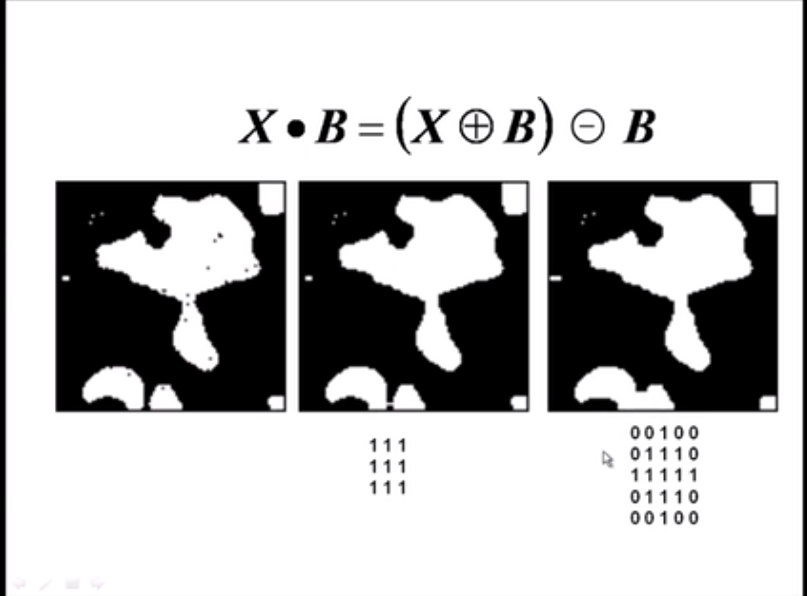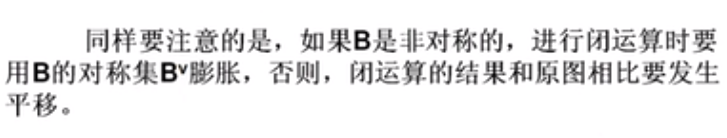## 开运算和闭运算的性质

X开运算的补集等于X补集的闭运算，或者X闭运算的补集等于X补集的开运算。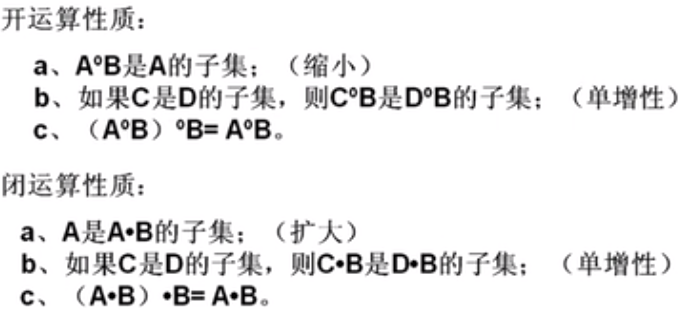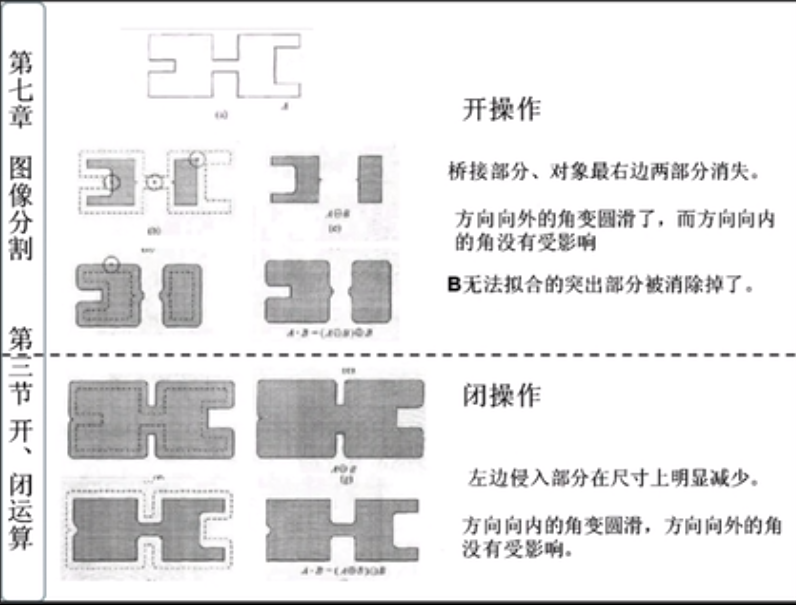# 二值数学形态学基本算法

## 形态滤波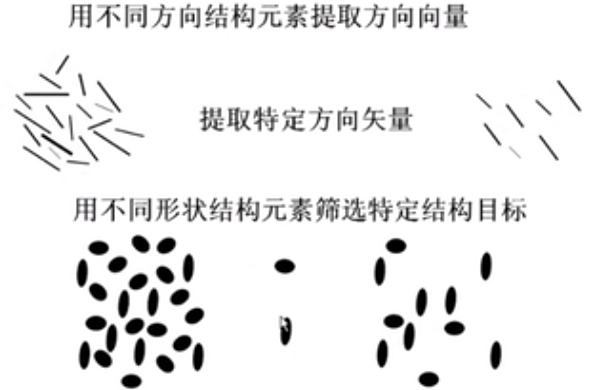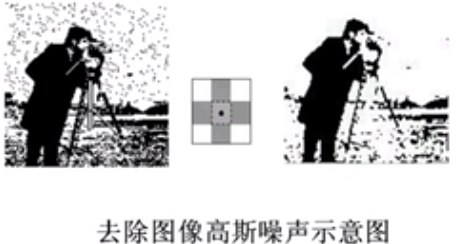## 图像的边缘提取

$Y=X-(X\circleddash B)$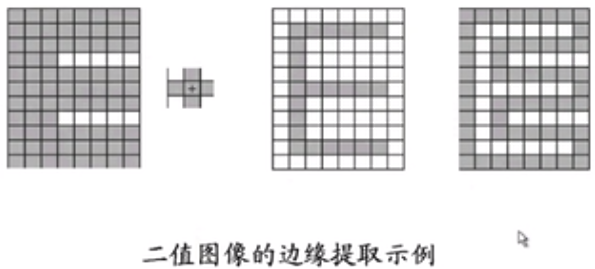## 区域填充

$X_k=(X_{k-1} \oplus B)\cap A^c$
B是结构元素，$X_0$是边界种子点，A是边界图像。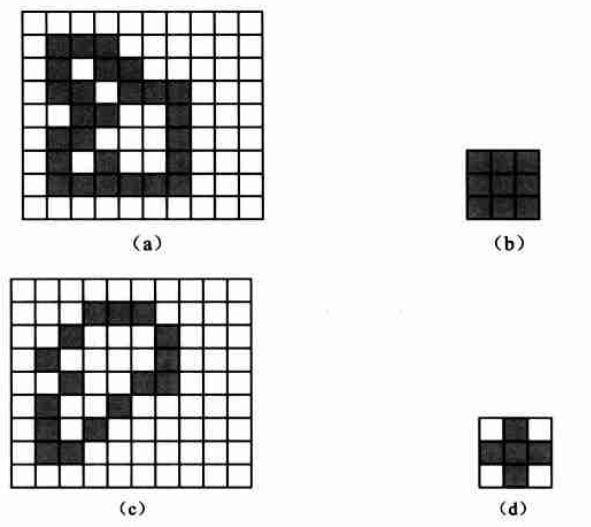## 击中击不中变换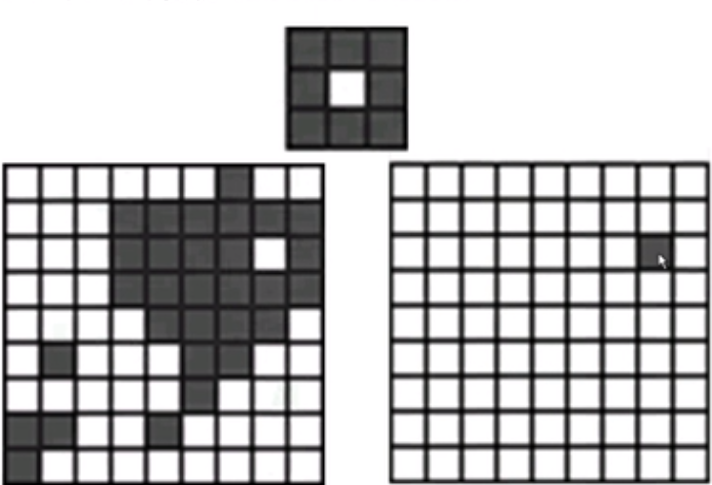2019-07-18 14:25:35 YueYingGuang 阅读数 3307
• ###### MATLAB图像处理

全面系统的学习MATLAB在图像处理中的应用

19905 人正在学习 去看看 魏伟

# MATLAB图像处理（一）——计算机图形学之图像形状识别

## 基本步骤：

### 3、确定所需形状的目标；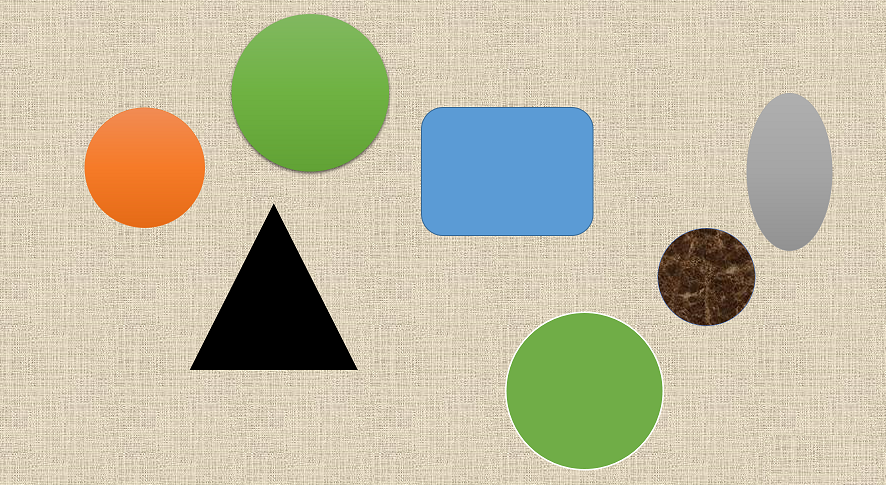## 1、读取彩色图像转化为二值图像

1） 读取彩色图像

% 1、读取图像并转化为二值图像
figure;imshow(RGB);title('原图像');


2）将彩色图像转化为二值图像

% 转化为灰度图像
I = rgb2gray(RGB);
% 设置阈值
threshold = graythresh(I);
% 转化为二值图像
bw = im2bw(I,threshold);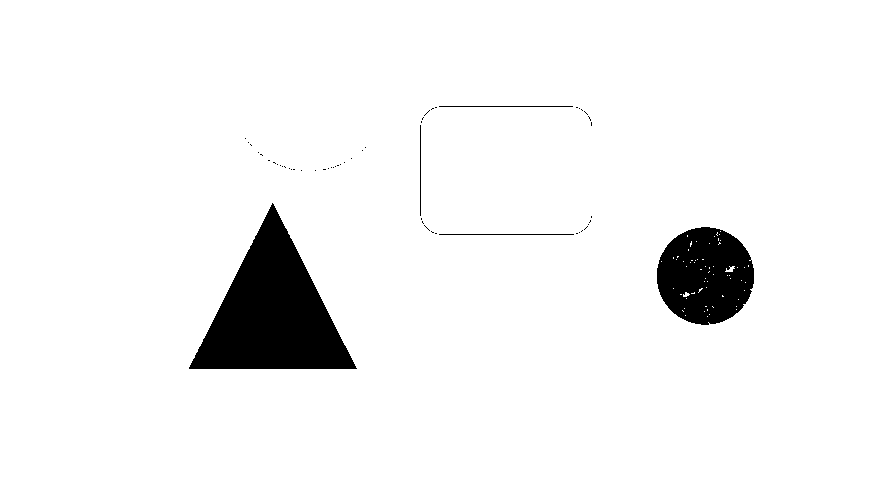bw = im2bw(I,0.69);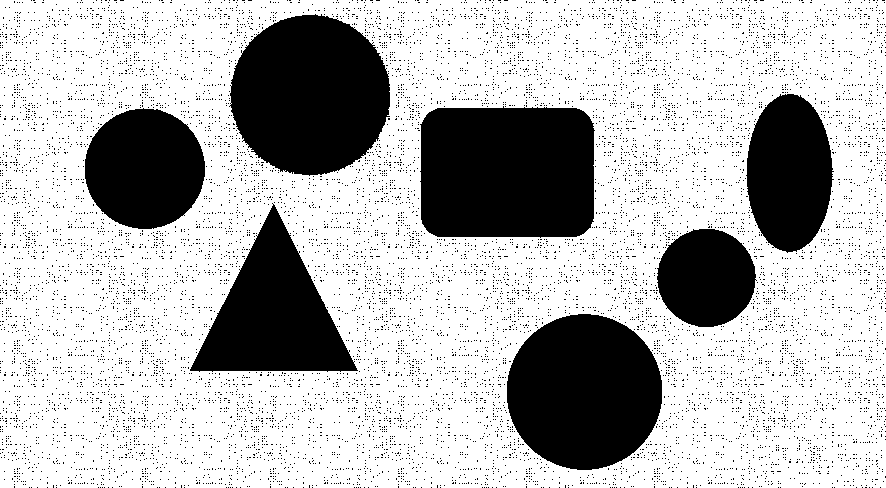% 通过领域判断手动去噪
[m,n] = size(bw);
for i = 2:m-1
for j = 2:n-1
%同上下元素判断
if(bw(i,j)~=bw(i+1,j) && bw(i,j)~=bw(i-1,j))
bw(i,j) = 1;
%同左右元素判断
elseif(bw(i,j)~=bw(i,j+1) && bw(i,j)~=bw(i,j-1))
bw(i,j) = 1;
%同斜边元素判断
elseif(bw(i,j)~=bw(i+1,j+1) && bw(i,j)~=bw(i-1,j-1))
bw(i,j) = 1;
%同斜边元素判断
elseif(bw(i,j)~=bw(i-1,j+1) && bw(i,j)~=bw(i+1,j-1))
bw(i,j) = 1;
end
end
end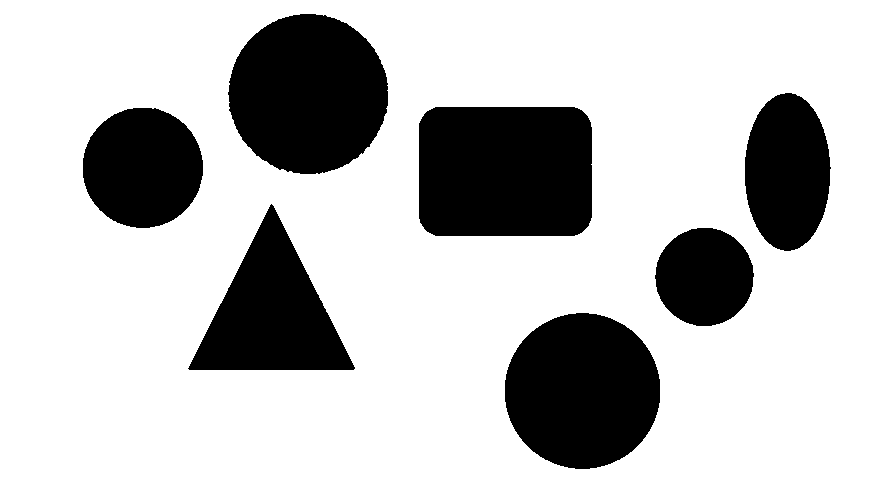for i = 1:m
for j = 1:n
bw(i,j) = ~bw(i,j);
end
end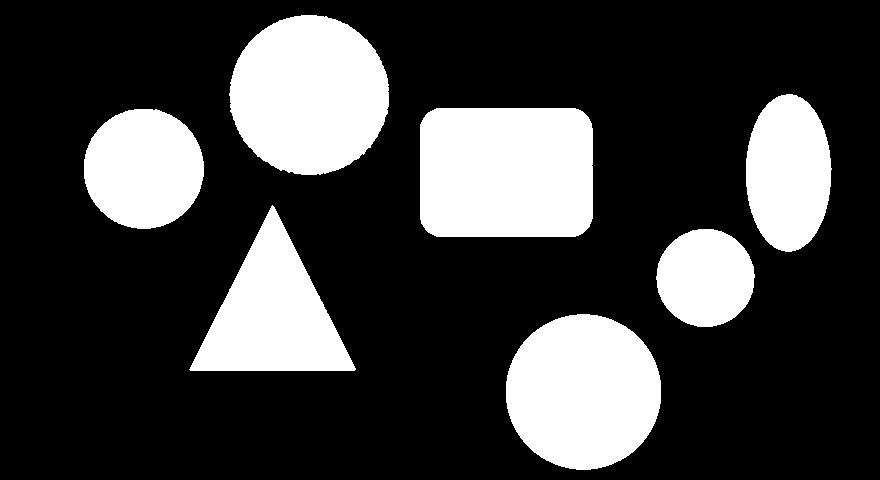## 2、确定图像中的形状边界

% 去除小目标，因为本图没有小目标，所以可以不需要本条语句
bw = bwareaopen(bw,30);
% 图形学结构元素构建，圆形
se = strel('disk',8);
% 关操作
bw = imclose(bw,se);
% 填充孔洞
bw = imfill(bw,'holes');
% 二值化图像显示
figure(1);imshow(bw);title('二值图像');
[B,L] = bwboundaries(bw,'noholes');
figure(2);imshow(label2rgb(L,@jet,[.5 .5 .5]));
hold on;
for k = 1:length(B)
boundary = B{k};
% 显示白色边界
plot(boundary(:,2),boundary(:,1),'w','LineWidth',2)
end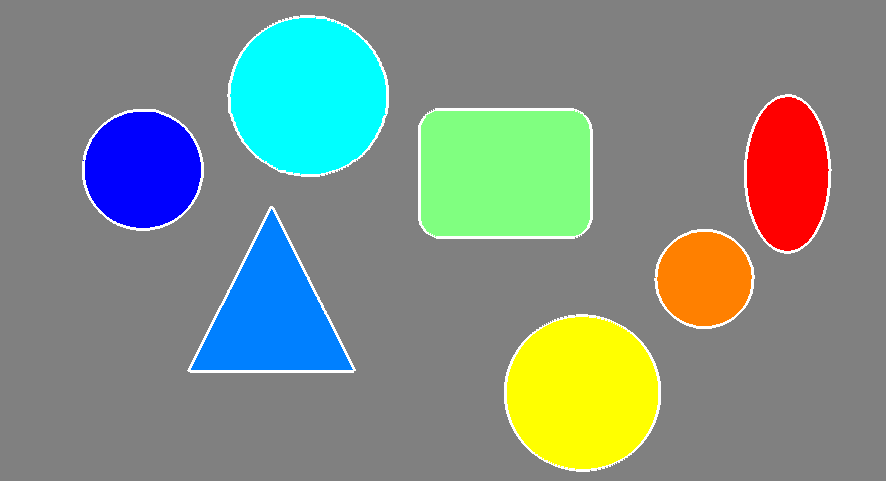## 3、确定所需形状的目标

hold on;
% 确定圆形目标
stats = regionprops(L,'Area','Centroid');
% 设置求面积
threshold = 0.85;
for k = 1:length(B)
boundary = B{k};
delta_sq = diff(boundary).^2;
% 求周长
perimeter = sum(sqrt(sum(delta_sq,2)));
% 求面积
area = stats(k).Area;
metric = 4*pi*area/perimeter^2;
metric_string = sprintf('%2.2f',metric);
% 根据阈值匹配
if metric > threshold
centroid = stats(k).Centroid;
plot(centroid(1),centroid(2),'ko');
text(centroid(1)-2,centroid(2)-2, '这是圆形','Color',...
'k','FontSize',14,'FontWeight','bold');
end
text(boundary(1,2)-10,boundary(1,1)-12, metric_string,'Color',...
'k','FontSize',14,'FontWeight','bold');
end
title('图像形状识别')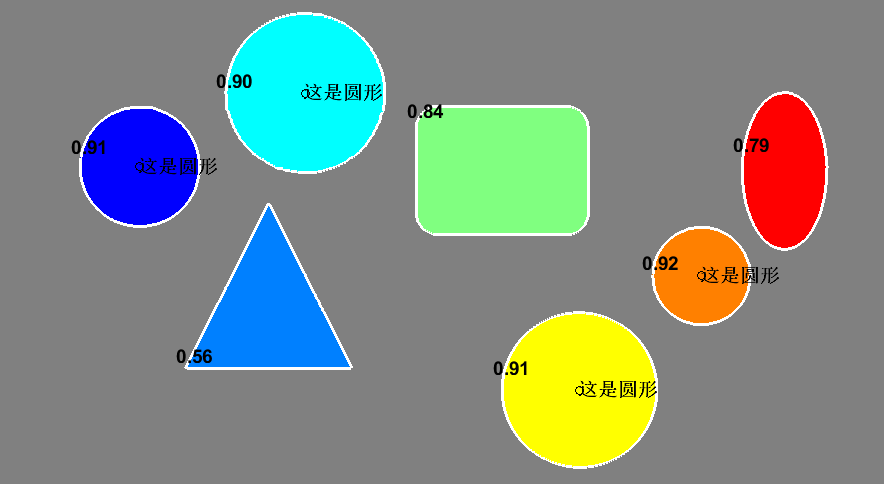2019-06-08 09:20:06 lin1094201572 阅读数 76
• ###### MATLAB图像处理

全面系统的学习MATLAB在图像处理中的应用

19905 人正在学习 去看看 魏伟

《图像处理》复习内容

1.图像传感器

2.数字化扫描

3.光电转换设备

3.合成图像

#### 5、图像的采样和量化

1.采样：将图像在空间（x,y)上离散化称为采样

2.量化：将采样后的图像离散为像素

#### 7、傅里叶变换

$F(u) = \int_{- \infty}^{+\infty}f(x)e^{-j 2 \pi u x}dx$

$f(x) = \int_{-\infty}^{+\infty}F(u)e^{j2\pi ux}du$

$F(u) = \int_{- \infty}^{+\infty}\int_{- \infty}^{+\infty}f(x)e^{-j 2 \pi (ux+uy)}dx$

$f(x) = \int_{- \infty}^{+\infty}\int_{- \infty}^{+\infty}F(u)e^{-j 2 \pi (ux+uy)}du$

$F(u)=\sum_{x=0}^{N-1}f(x)e^{-j\frac{2\pi ux}{N}}$

$f(x)=\frac{1}{N}\sum_{u=0}^{N-1}F(u)e^{-j\frac{2\pi ux}{N}}$

1.线性特性 2.比例特性 3.平移性质 4.可分离性 5.周期性 6.共轭对称性 7.旋转不变性 8.微分性 9平均值性质 10.卷积定理 11.相关定理 12.帕沙瓦（Parseval)定理（能量不变)

$F(u,v)=\sum_{x=0}^{M-1}\sum_{y=1}^{N-1}f(x,y)e^{-j2\pi(\frac{ux}{M}+\frac{vy}{N})}$

$f(x,y)=\frac{1}{MN}\sum_{u=0}^{M-1}\sum_{v=1}^{N-1}F(u,v)e^{-j2\pi(\frac{ux}{M}+\frac{vy}{N})}$

#### 8、离散余弦变换

DFT(离散傅里叶变换)是频谱分析的有力工具，但DFT是基于复数域的运算，因而给实数运算带来不变。

DCT变换具有正交变换的性质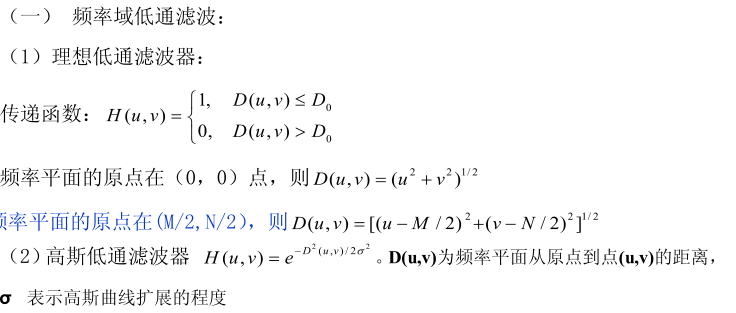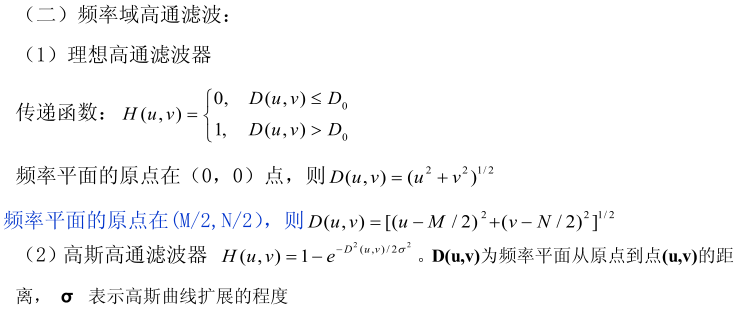1）空域增强

1.1.灰度变换

1.1.1直接灰度变换

1.1.2直方图灰度变换

1.1.3图像代数运算

1.2.空域滤波

1.2.1平滑滤波

1.2.2锐化滤波

2）频域增强

2.1 低频滤波

2.2 高通滤波

2.3 带通滤波

2.4 带阻滤波

#### 11、直方图的图像增强

1.首先对原始图像进行直方图均衡化处理

2.依据原始图像均衡化后的图像的灰度值得到目标图像的灰度级z

12、空域滤波

13、低通滤波#### 14、高通滤波16、无约束复原技术

17、逆滤波器

18、几何畸变校正

19、盲目图像复原

1）直接测量法 2)间接估计法

20、图像编码的基本原理

21、图像统计编码

22、预测编码

23、图像分割的基本概念

24、阀值分割

25、区域分割

26、边缘检测

27、几何特征

28、颜色特征

29、纹理特征

#### 30、骨架的概念

2016-01-04 14:52:41 lx407655880 阅读数 140
• ###### MATLAB图像处理

全面系统的学习MATLAB在图像处理中的应用

19905 人正在学习 去看看 魏伟

1.通过坐标变换矩阵 去改变图像的形状大小位置等 （9位 float）A  B C

D E F

G H I                          --> AE控制缩放，CF控制平移， BD控制错切

2.  android API操作

matrix.setRote(); -- 旋转

matrix.setTranslate() -- 平移

matrix.setScale() -- 缩放

matrix.setSkew() -- 错切

post -- 矩阵组合

3.     通过画笔风格实现不同图形特效   -Xfermode

PorterDuff.Mode为枚举类,一共有16个枚举值:

1.PorterDuff.Mode.CLEAR

所绘制不会提交到画布上。
2.PorterDuff.Mode.SRC

显示上层绘制图片
3.PorterDuff.Mode.DST

显示下层绘制图片
4.PorterDuff.Mode.SRC_OVER

正常绘制显示，上下层绘制叠盖。
5.PorterDuff.Mode.DST_OVER

上下层都显示。下层居上显示。
6.PorterDuff.Mode.SRC_IN

取两层绘制交集。显示上层。
7.PorterDuff.Mode.DST_IN

取两层绘制交集。显示下层。
8.PorterDuff.Mode.SRC_OUT

取上层绘制非交集部分。
9.PorterDuff.Mode.DST_OUT

取下层绘制非交集部分。
10.PorterDuff.Mode.SRC_ATOP

取下层非交集部分与上层交集部分
11.PorterDuff.Mode.DST_ATOP

取上层非交集部分与下层交集部分
12.PorterDuff.Mode.XOR

异或：去除两图层交集部分
13.PorterDuff.Mode.DARKEN

取两图层全部区域，交集部分颜色加深
14.PorterDuff.Mode.LIGHTEN

取两图层全部，点亮交集部分颜色
15.PorterDuff.Mode.MULTIPLY

取两图层交集部分叠加后颜色
16.PorterDuff.Mode.SCREEN

取两图层全部区域，交集部分变为透明色

public class RoundRectXfermodeView extends View {

private Bitmap mBitmap;
private Bitmap mOut;
private Paint mPaint;

public RoundRectXfermodeView(Context context) {
super(context);
initView();
}

public RoundRectXfermodeView(Context context, AttributeSet attrs) {
super(context, attrs);
initView();
}

public RoundRectXfermodeView(Context context, AttributeSet attrs, int defStyleAttr) {
super(context, attrs, defStyleAttr);
initView();
}

private void initView() {
setLayerType(LAYER_TYPE_SOFTWARE, null);    //    要禁用 硬件加速
mBitmap = BitmapFactory.decodeResource(getResources(), R.drawable.test1);
mOut = Bitmap.createBitmap(mBitmap.getWidth(),
mBitmap.getHeight(),
Bitmap.Config.ARGB_8888);
Canvas canvas = new Canvas(mOut);
mPaint = new Paint(Paint.ANTI_ALIAS_FLAG);
// Dst  遮罩层
canvas.drawRoundRect(0, 0, mBitmap.getWidth(), mBitmap.getHeight(),
50, 50, mPaint);
mPaint.setXfermode(new PorterDuffXfermode(PorterDuff.Mode.SRC_IN));  // 设置画笔的 xfermode属性为  src_in
// Src
canvas.drawBitmap(mBitmap, 0, 0, mPaint);
mPaint.setXfermode(null);
}

@Override
protected void onDraw(Canvas canvas) {
canvas.drawBitmap(mOut, 0, 0, null);
}
}

bitmap   在渲染器内使用的位图

tileX      The tiling mode for x to draw the bitmap in.   在位图上X方向渲染器平铺模式

tileY     The tiling mode for y to draw the bitmap in.    在位图上Y方向渲染器平铺模式

TileMode：

CLAMP  ：如果渲染器超出原始边界范围，会复制范围内边缘染色。

REPEAT ：横向和纵向的重复渲染器图片，平铺。

MIRROR ：横向和纵向的重复渲染器图片，这个和REPEAT重复方式不一样，他是以镜像方式平铺。

import android.content.Context;
import android.graphics.Bitmap;
import android.graphics.Canvas;
import android.graphics.Paint;
import android.graphics.drawable.BitmapDrawable;
import android.graphics.drawable.ShapeDrawable;
import android.graphics.drawable.shapes.OvalShape;
import android.util.AttributeSet;
import android.view.View;

public class BitmapShaderView extends View {

private Bitmap bitmap = null;
private Paint paint = null;
private ShapeDrawable shapeDrawable = null;
private int BitmapWidth = 0;
private int BitmapHeight = 0;

super(context);

// 得到图像
bitmap = ((BitmapDrawable) getResources().getDrawable(R.drawable.cat))
.getBitmap();
BitmapWidth = bitmap.getWidth();
BitmapHeight = bitmap.getHeight();
}

super(context, set);
}

@Override
protected void onDraw(Canvas canvas) {
// TODO Auto-generated method stub
super.onDraw(canvas);
//将图片裁剪为椭圆形
//构建ShapeDrawable对象并定义形状为椭圆
shapeDrawable = new ShapeDrawable(new OvalShape());
//得到画笔并设置渲染器
//设置显示区域
shapeDrawable.setBounds(20, 20,BitmapWidth-140,BitmapHeight);
//绘制shapeDrawable
shapeDrawable.draw(canvas);
}

}

5. 像素块   drawBitmapMesh

public class MeshView extends View {

private int WIDTH = 200;
private int HEIGHT = 200;
private int COUNT = (WIDTH + 1) * (HEIGHT + 1);
private float[] verts = new float[COUNT * 2];
private float[] orig = new float[COUNT * 2];
private Bitmap mBitmap;
private float K = 1;

public MeshView(Context context) {
super(context);
initView();
}

public MeshView(Context context, AttributeSet attrs) {
super(context, attrs);
initView();
}

public MeshView(Context context, AttributeSet attrs, int defStyleAttr) {
super(context, attrs, defStyleAttr);
initView();
}

private void initView() {
int index = 0;
mBitmap = BitmapFactory.decodeResource(getResources(), R.drawable.test);
float bmWidth = mBitmap.getWidth();
float bmHeight = mBitmap.getHeight();

for (int i = 0; i < HEIGHT + 1; i++) {
float fy = bmHeight * i / HEIGHT;
for (int j = 0; j < WIDTH + 1; j++) {
float fx = bmWidth * j / WIDTH;
orig[index * 2 + 0] = verts[index * 2 + 0] = fx;
orig[index * 2 + 1] = verts[index * 2 + 1] = fy + 200;
index += 1;
}
}
}

@Override
protected void onDraw(Canvas canvas) {
for (int i = 0; i < HEIGHT + 1; i++) {
for (int j = 0; j < WIDTH + 1; j++) {
verts[(i * (WIDTH + 1) + j) * 2 + 0] += 0;
float offsetY = (float) Math.sin((float) j / WIDTH * 2 * Math.PI + K * 2 * Math.PI);
verts[(i * (WIDTH + 1) + j) * 2 + 1] =
orig[(i * (WIDTH + 1) + j) * 2 + 1] + offsetY * 50;
}
}
K += 0.1F;
canvas.drawBitmapMesh(mBitmap, WIDTH, HEIGHT,
verts, 0, null, 0, null);
invalidate();
}
}